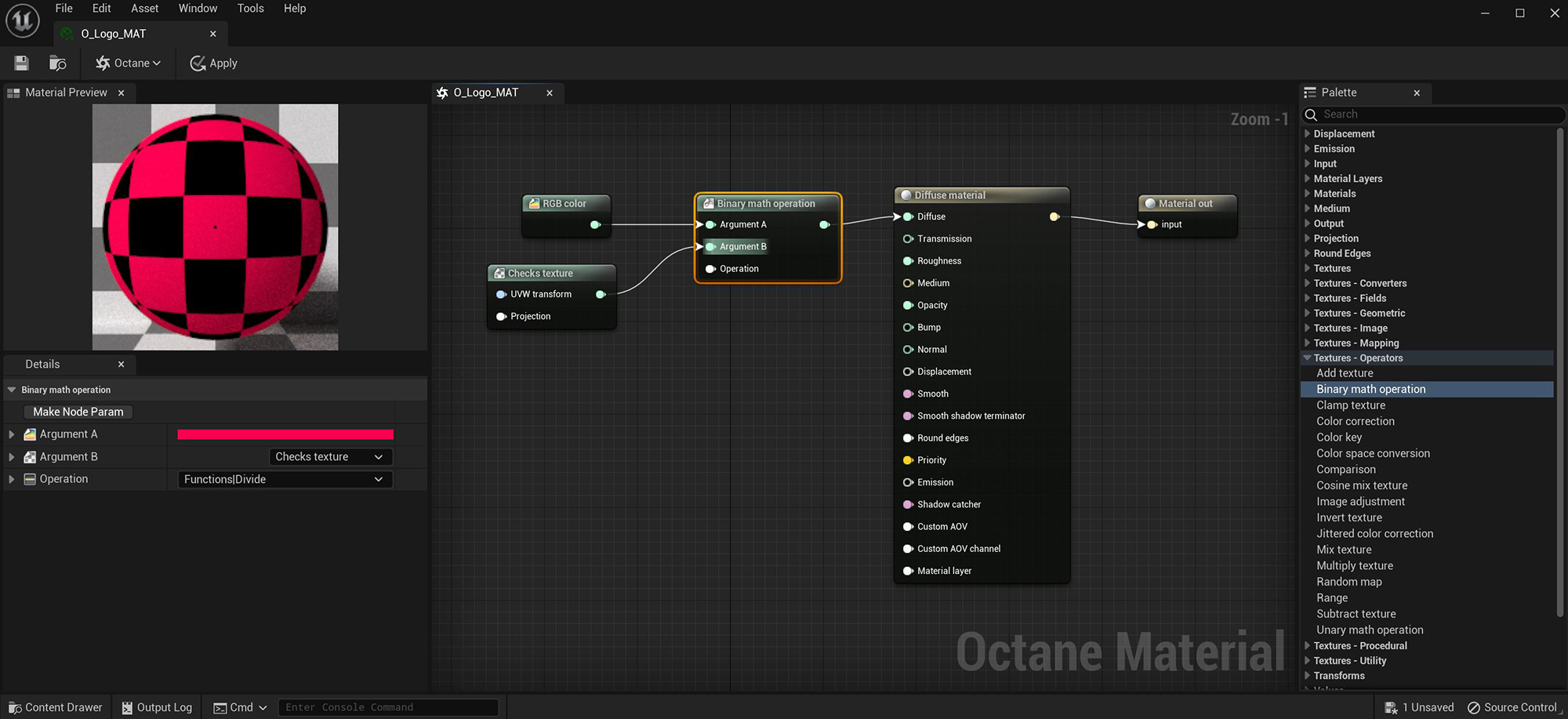# Binary Math Operation

The Binary Math node takes two inputs as operands for various mathematical calculations determined in the Operation parameter (figure 1).Figure 1: A RGB Color node and a Checks Texture node are blended together using the Divide Operation, resulting in a red and black checker pattern

## Binary Math Operation Parameters

Argument A - The first argument for the operation.

Argument B - The second argument for the operation.

Operation - The calculation to be performed on the arguments.